# Plastic Hinge Formation in a Beam Structure

Problem Statement

Note

To view this project in FLAC3D, use the menu command Help ► Examples…. Choose “Structure/Beam/PlasticHinge” and select “PlasticHinge.prj” to load. The main data files used are shown at the end of this example. The remaining data files can be found in the project.

This example demonstrates two methods, referred to as the single-node and the double-node methods, by which FLAC3D can be used to calculate the initiation and subsequent behavior of a plastic hinge in a beam. The single-node method involves specifying the limiting plastic moment that can be carried by the elements making up the beam. This is done by specifying the desired plastic-moment capacity using the structure beam property plastic-moment command. With this method, a hypothetical hinge can form between beam elements; however, because the elements are joined with a single node, a true discontinuity in the rotational motion cannot develop at these locations. The double-node method involves creating double nodes at each potential hinge location and then appropriately linking these nodes together. The double nodes allow a discontinuity in the rotation to occur when the limiting plastic moment is reached. The double-node method should be applied to calculate the large-strain, post-failure behavior of a structure. If it is only necessary to determine the solution at the limiting plastic moment, then the single-node method is sufficient. In the following discussion, we first define the example problem, and then solve it in two ways by using the single-node method, and then using the double-node method.

Single Node

A concentrated vertical load, $$P$$, is applied at the center of a 10 m long, simply supported beam with a plastic-moment capacity, $$M^P$$, of 25 kN-m. The system, along with the shear and moment diagrams, is shown in Figure 1. From these shear and moment diagrams, we find that the specified plastic-moment capacity corresponds with a maximum vertical load of 10 kN and a maximum shear force of 5 kN. If we apply a constant vertical velocity to the beam center, we expect that the limiting values of moment and shear force will be 25 kN-m and 5 kN, respectively.Figure 1: Single beam with single concentrated load.

The FLAC3D model demonstrating the single-node method (see “PlasticHinge-Single.dat”) is created by issuing a single structure beam create command and specifying two segments. This produces a model containing 2 elements and 3 nodes, with both elements sharing the center node (node ID of 3). The beam is assigned the same properties as in the Concentrated Loads example but, in addition, the plastic-moment capacity is set to 25 kN-m with the structure beam property plastic-moment command. Simple supports are specified at the beam ends by restricting translation in the $$y$$-direction. A constant vertical velocity is applied to the center node, and the moment and shear force acting at the right end of element 1 are monitored during the calculation to determine when the limiting value is reached. Note that we specify combined local damping for this problem (structure damping combined-local) in order to eliminate the ringing that can occur with the default local damping scheme when the system is being driven by a constant motion.

We find that the limiting values of moment and shear force are equal to the analytical values of 25 kN-m and 5 kN, respectively (see Figure 2 and Figure 3). Also, the moment and shear force distributions correspond with the analytical solution (see Figure 4 and Figure 5). We also see that a discontinuity in the rotational motion at the center location does not develop; the rotation of the center node is zero (structure node list displacement).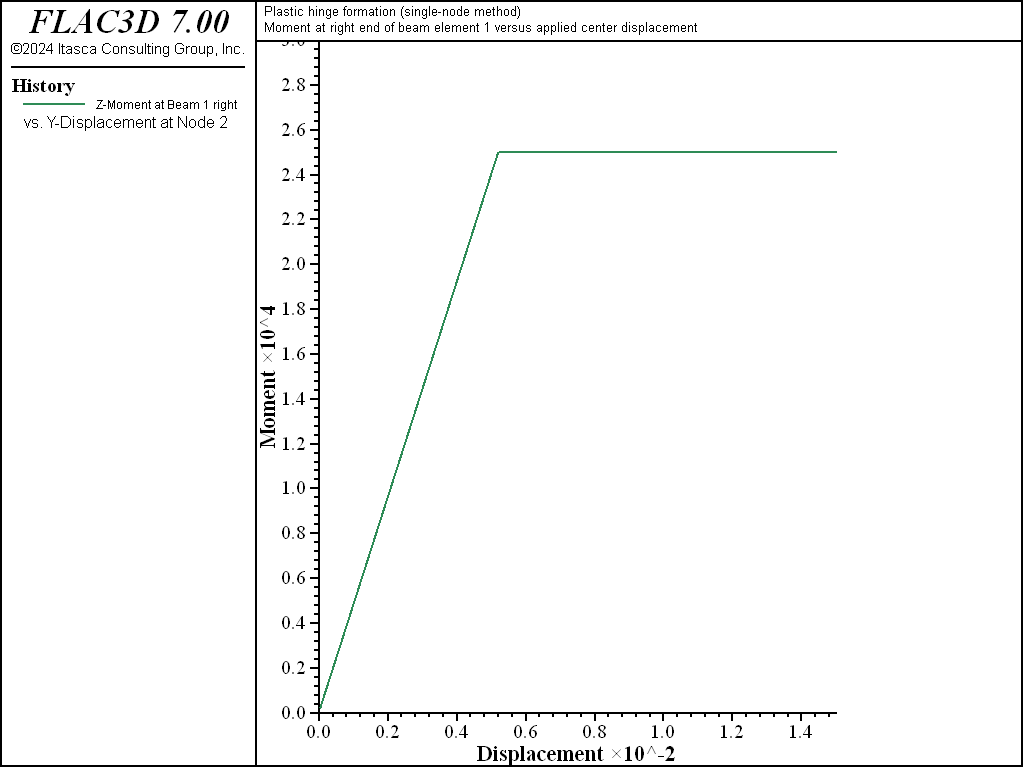Figure 2: Moment at right end of element 1 versus applied center displacement (single-node method).Figure 3: Shear force at right end of element 1 versus applied center displacement (single-node method).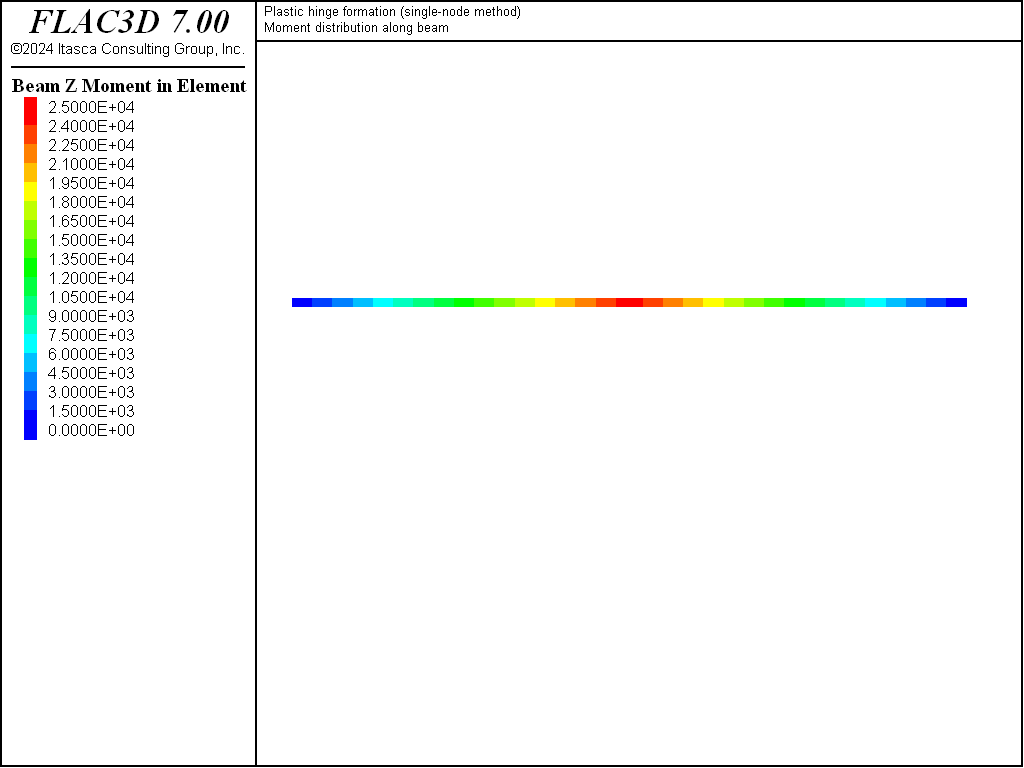Figure 4: Moment distribution at limit condition (single-node method).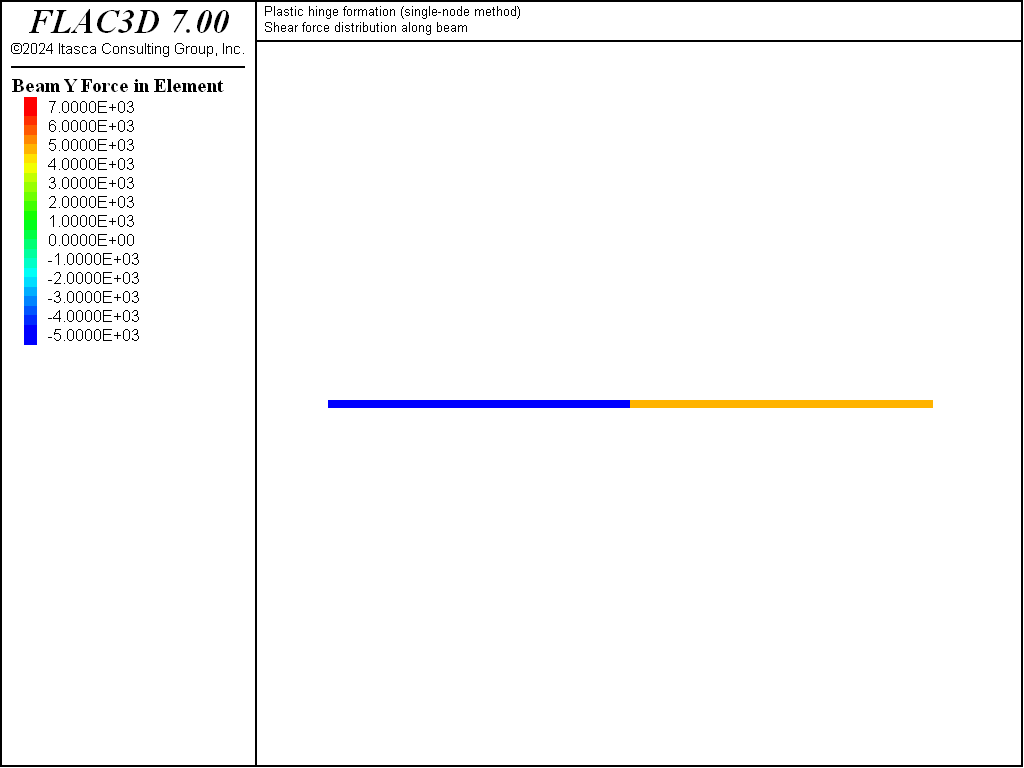Figure 5: Shear force distribution at limit condition (single-node method).

Double Node

The FLAC3D model demonstrating the double-node method (see “PlasticHinge-Double.dat”) is created by issuing two separate structure beam create commands. This produces a model containing two elements and four nodes. Going from left to right, the left element uses nodes 1 and 2, and the right element uses nodes 3 and 4. Note that nodes 2 and 3 lie in the same location, but they will not interact with one another. We now create an appropriate linkage between nodes 2 and 3 with the commands

struct node join
struct link property rotation-z area=1.0 stiffness=6.4e8 yield-compression=25e3 yield-tension=25e3


The first command creates a node-to-node link from node 2 to node 3. The second command changes the default attachment condition in the $$z$$-rotational direction from rigid to deformable. The third command sets the properties of this normal-yield spring as follows. We set the area to unity, and we set both the compressive and tensile yield strengths equal to the desired plastic-moment capacity of 25 kN-m. Finally, we set the spring stiffness equal to a value that is large enough to make the spring deformation small relative to the beam deformation. We determine this value by computing the rotational stiffnesses of the two elements that use the spring (each rotational stiffness is $$4EI/L$$, where $$L$$ is element length) and multiplying this value by 10.

Now that the double nodes have been appropriately linked to one another, the beam is assigned the same properties as in Concentrated Loads—we do not specify a plastic-moment capacity, because we want the plastic moment to develop in the normal-yield spring. Simple supports are specified at the beam ends by restricting translation in the $$y$$-direction. A constant vertical velocity is applied to the center nodes (the applied velocity of the node with the rigid attachment condition is ignored) and the moment and shear force acting at the right end of element 1 are monitored during the calculation to determine when the limiting value is reached. Note that we specify combined local damping for this problem (structure damping combined-local) in order to eliminate the ringing that can occur with the default local damping scheme when the system is being driven by a constant motion.

We find that the limiting values of moment and shear force are equal to the analytical values of 25 kN-m and 5 kN, respectively (see Figure 6 and Figure 7). Also, the moment and shear force distributions correspond with the analytical solution (see Figure 8 and Figure 9). We also see that a discontinuity in the rotational motion at the center location has developed; the rotation of nodes 2 and 3 are nonzero and equal and opposite to each other (structure node list displacement).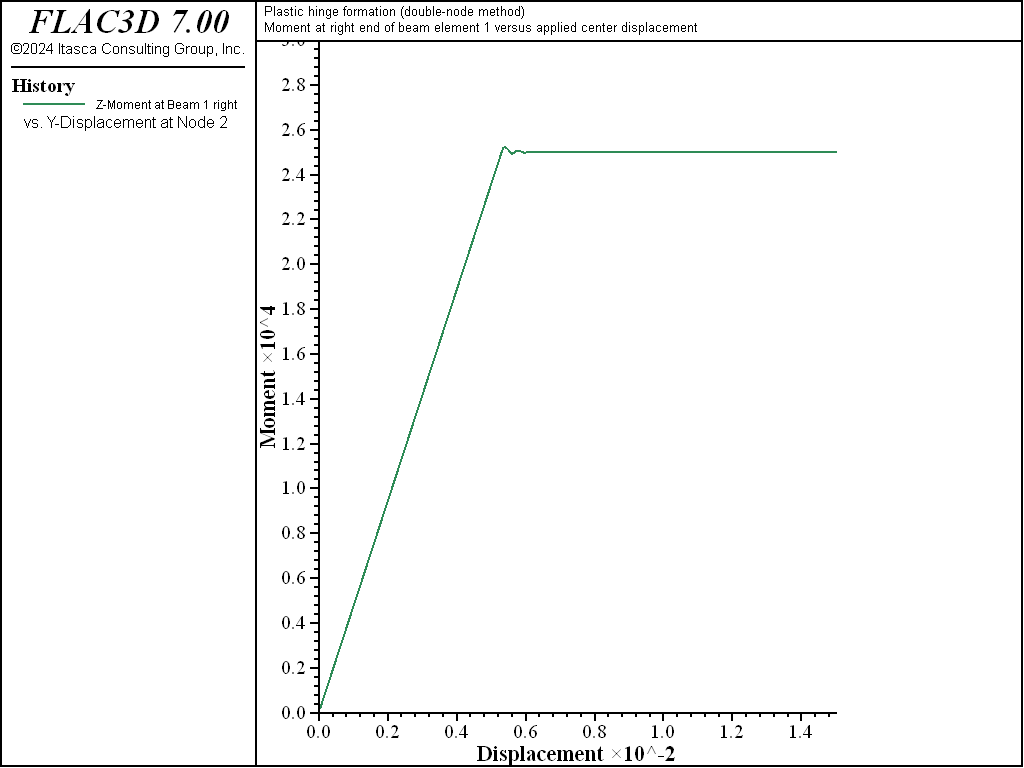Figure 6: Moment at right end of element 1 versus applied center displacement (double-node method).Figure 7: Shear force at right end of element 1 versus applied center displacement (double-node method).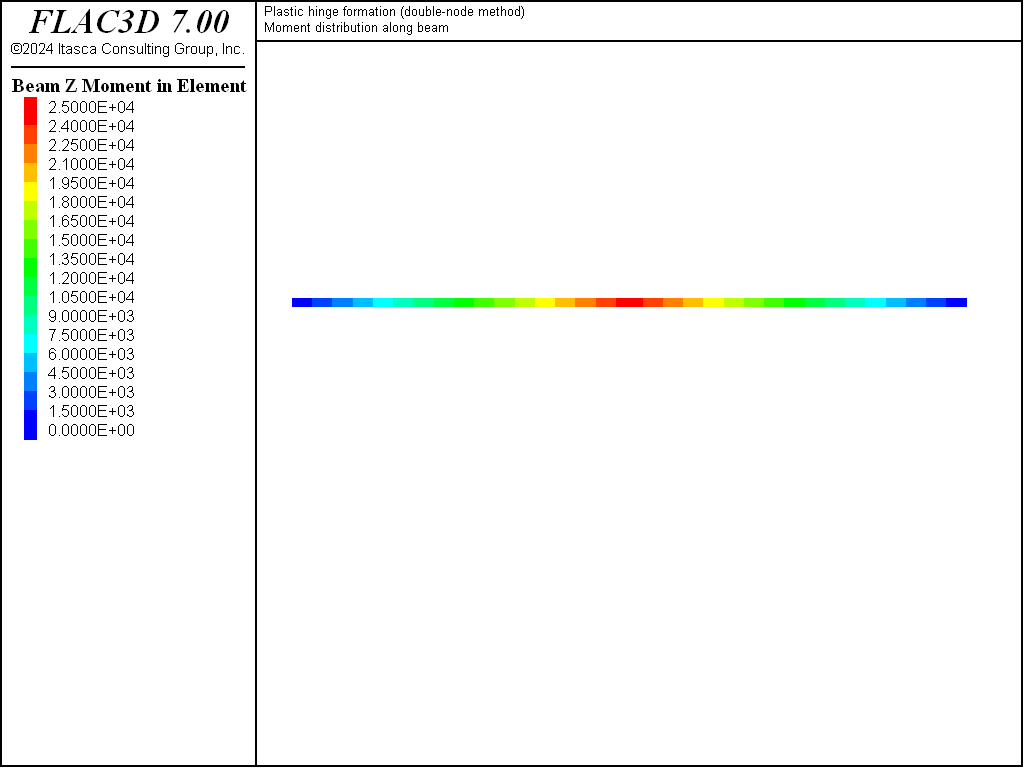Figure 8: Moment distribution at limit condition (double-node method).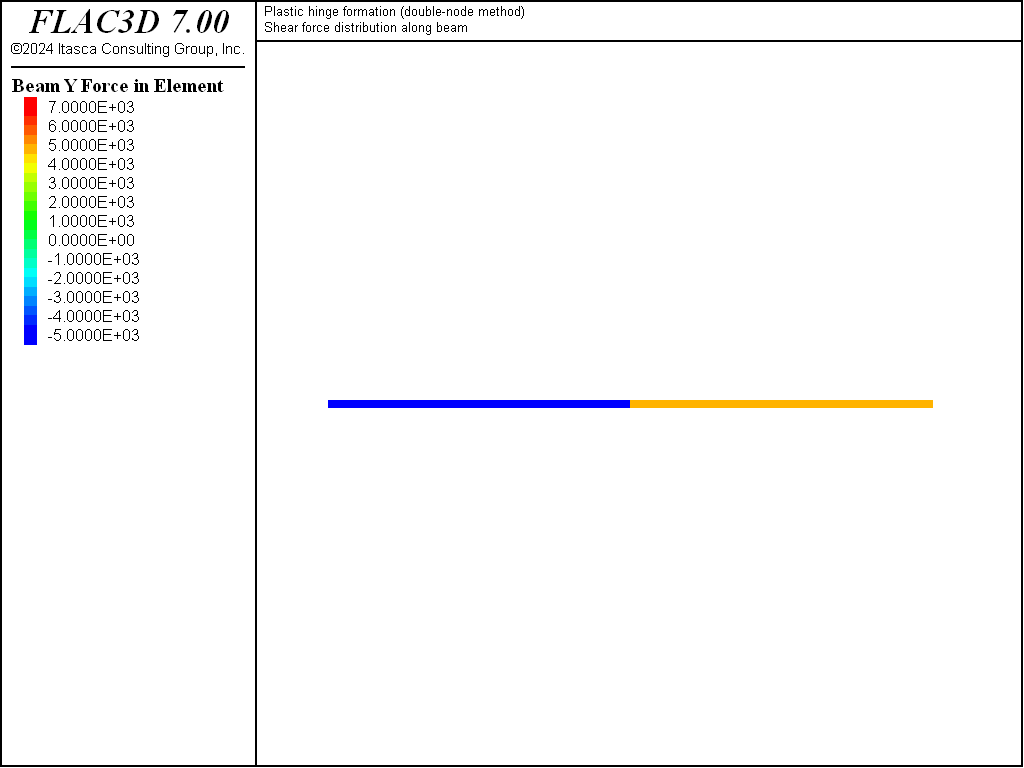Figure 9: Shear force distribution at limit condition (double-node method).

Double Node Cantilever

These two examples demonstrate that both the single-node and the double-node methods produce the same behavior near the limiting moment; however, if we were to continue loading the structure in a large-strain fashion, then the double-node method would produce more reasonable results because it would allow a discontinuity to develop in the rotation at the center. We illustrate this behavior by modifying the double-node example to represent a cantilever beam (fixed at the left end) with a vertical load applied at the free end (see “PlasticHinge-Cantilever.dat”). The problem is run in large-strain mode, and the load is specified such that it will remain oriented in the negative $$y$$-direction throughout the simulation.

The final structural configuration and moment distribution are shown in Figure 10. We see that the double-node method allows a discontinuity to develop in the rotation at the beam center.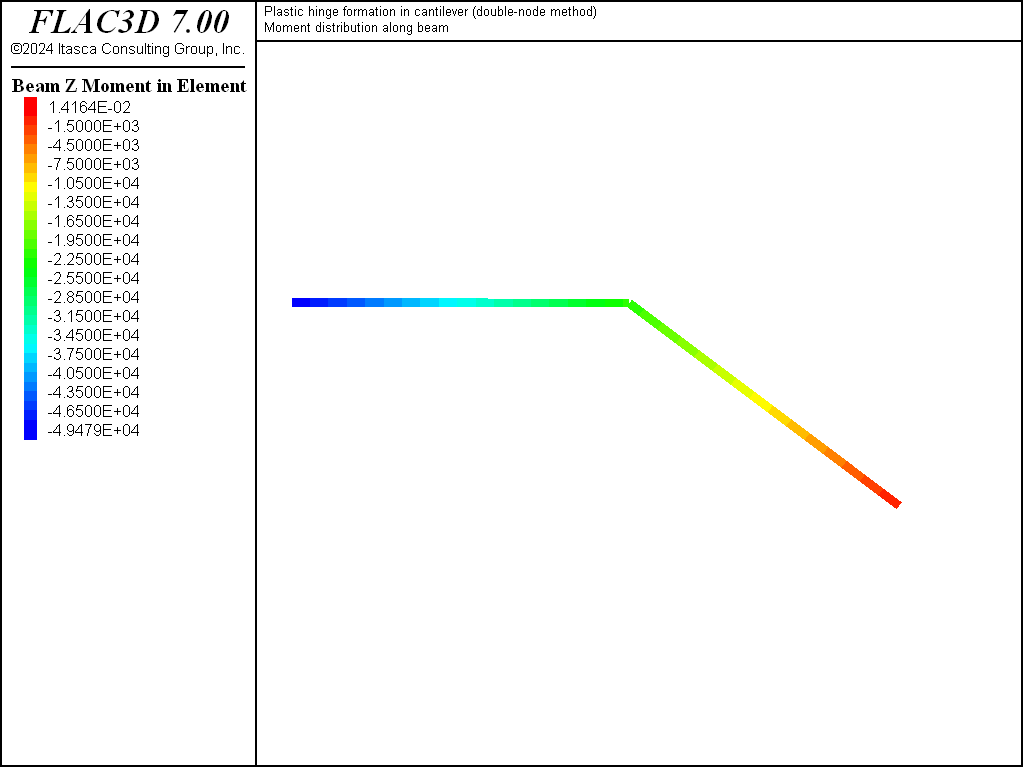Figure 10: Final structural configuration and moment distribution in cantilever (double-node method).

Data Files

PlasticHinge-Single.dat

model new
model large-strain off
model title "Plastic hinge formation (single-node method)"
; Create beam and assign properties
struct beam create by-line (0,0,0) (10,0,0) segments=2
struct beam property young=2.0e11 poisson=0.30
struct beam property cross-sectional-area=6e-3 moi-z=200e-6 ...
moi-y=200e-6 moi-polar=0.0
struct beam property plastic-moment=25e3
; Assign boundary conditions
struct node fix velocity-z rotation-x rotation-y
; restrict all non-beam modes
struct node fix velocity-y range union position-x 0.0 position-x 10
; simple support at left and right end
struct node fix velocity-y range position-x 5
; apply constant vertical velocity
struct node initialize velocity-y -5e-6 local range position-x 5
; to center node
; Take histories
struct node history name='disp'  displacement-y position (5,0,0)
; y-displacement of center node
struct beam history name='mom'   moment-z end 2 position (2.5,0,0)
; moment
struct beam history name='force' force-y end 2 position (2.5,0,0)
; shear force
; Set damping, and cycle
struct mechanical damping combined-local
model cycle 3000 ; .015 total displacmeent
model save 'Single'


PlasticHinge-Double.dat

; File for Plastic hinge formation (double-node method)
model new
model large-strain off
model title "Plastic hinge formation (double-node method)"
; Create beams
struct beam create by-line (0,0,0) (5,0,0)  id=1 segments=1
struct beam create by-line (5,0,0) (10,0,0) id=2 segments=1
; Join nodes, and make the z rot link plastic
struct node join
struct link property rotation-z area=1.0 stiffness=6.4e8 ...
yield-compression=25e3 yield-tension=25e3
; Assign beam properties
struct beam property young=2.0e11 poisson=0.30
struct beam property cross-sectional-area=6e-3 moi-z=200e-6 ...
moi-y=200e-6 moi-polar=0.0
; Assign beam boundary conditions
struct node fix velocity-z rotation-x rotation-y
; restrict all non-beam modes
struct node fix velocity-y range union position-x 0.0 position-x 10
; simple support at left and right end
; apply constant vertical velocity to center node-3 ---
; must apply velocity to target node.
struct node fix velocity-y range position-x 5
; apply constant vertical velocity
struct node initialize velocity-y -5e-6 local range position-x 5
; to center node
; Take histories
struct node history name='disp'  displacement-y position (5,0,0)
; y-displacement of center node
struct beam history name='mom'   moment-z end 2 position (2.5,0,0)
; moment
struct beam history name='force' force-y end 2 position (2.5,0,0)
; shear force
; Set damping and cycle
struct mechanical damping combined-local
model cycle 3000 ; .015 total displacement
model save 'Double'


PlasticHinge-Cantilever.dat

; file for Plastic hinge formation in cantilever (double-node method)
model new
model title "Plastic hinge formation in cantilever (double-node method)"
; Create two beams
struct beam create by-line (0,0,0) (5,0,0)  id=1 segments=1
struct beam create by-line (5,0,0) (10,0,0) id=2 segments=1
; Join nodes, and make the z rot link plastic
struct node join
struct link property rotation-z area=1.0 stiffness=6.4e8 ...
yield-compression=25e3 yield-tension=25e3
; Assign beam properties
struct beam property young=2.0e11 poisson=0.30
struct beam property cross-sectional-area=6e-3 moi-z=200e-6 ...
moi-y=200e-6 moi-polar=0.0
; Assign boundary conditions
struct node fix velocity-z rotation-x rotation-y ; restrict all non-beam modes
struct node fix velocity rotation range position-x=0      ; fully fix left end
struct node apply force=(0,-5.5e3,0) range position-x=10  ; apply force at tip
; Assign damping, and cycle
struct mechanical damping combined-local
model large-strain on
model solve ratio-local 1e-4
model save 'Cantilever'